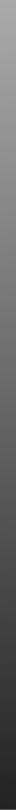Overview
Forum
Mango
iMango
Papaya
Multi-image Analysis GUI

## Find Principal Axes Plugin

Preprocessing:

1. Within Mango define ROIs of interest. Mango supports eight ROIs, each determined by a different color.
2. From the Plugins menu select Find Principal Axes.

Principal Axes Plugin Use:

1. Select ROI by color and press Analyze.
2. Change to other ROIs if necessary and press Analyze.
3. Results are printed one after the other to a scrolling window, and text can be copied for use elsewhere.

Results:

1. The Principal Axes Plugin identifies each ROI by color name at the top of its results.
2. General summary statistics follow including centroid, voxel volume, and volume in mm3.
3. Next is the raw covariance matrix.
4. Next is the diagonalized covariance matrix with eigenvalues along its diagonal elements. These are arranged from smallest at upper left to largest at lower right.
5. Last are the eigenvectors in column format. The x, y and z coordinates are from top to bottom row. The leftmost column eigenvector corresponds to the leftmost eigenvalue and so forth. Each eigenvector is unit length.

Interpretation:

1. Each eigenvalue is the mean square distance from the centroid to voxels within the ROI in the direction of its corresponding eigenvector. To convert this value to a distance measure you MUST take the square root of the eigenvalue.
2. The directed distances formed from the square root of the eigenvalues are along three principal axes with directions determined by the corresponding eigenvectors.
3. The coordinate system used for centroid are x,y,z with x=0 at left, y=0 at anterior, and z=0 at superior ends of the image volume.
4. The coordinate system used for covariance matrices and eigenvectors is referenced to the centroid with +x right, +y posterior, and +z inferior of the centroid. This is not a standard brain coordinate system, but was used to simplify processing.

Version History:
1.3 (06-05-2015) — Added script support.
1.2 (05-16-2014) — Changes to support plugin API updates.
1.1.3 (03-18-2014) — Minor change to support plugin API updates.
1.1.2 (01-24-2014) — Minor fix
1.1.1 — Can now resize window. Better support for multi-displays.
1.0.1 — Added support for new plugin format.